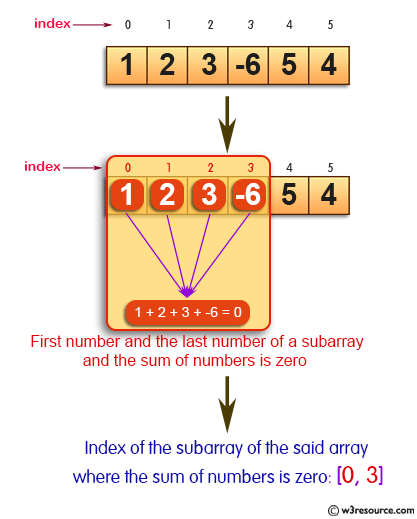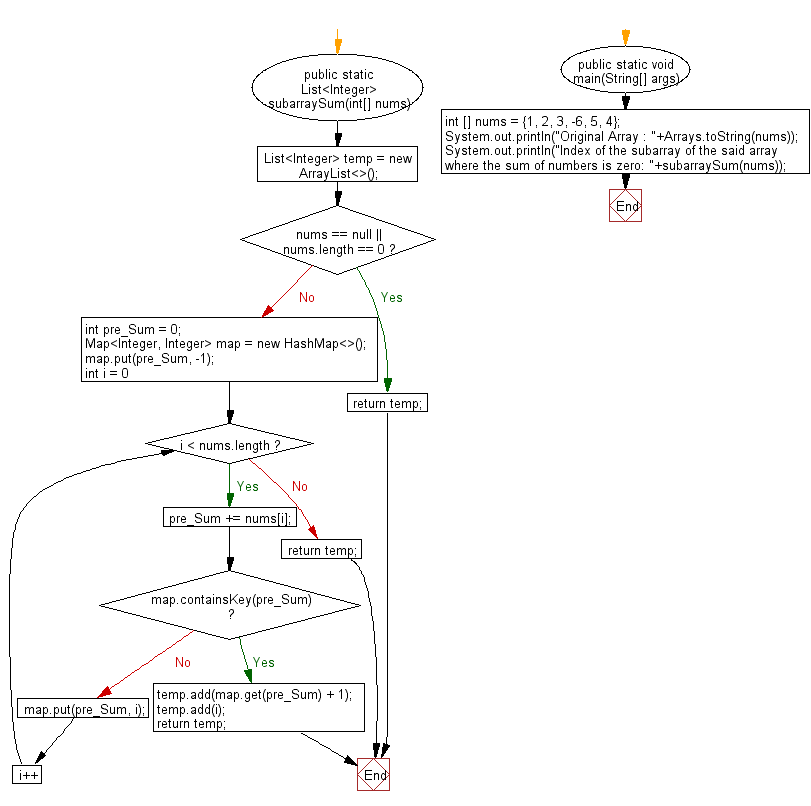﻿ Java: Index of the first and the last number of a subarray# Java Exercises: Get the index of the first number and the last number of a subarray

## Java Basic: Exercise-139 with Solution

Write a Java program to get the index of the first number and the last number of a subarray where the sum of numbers is zero from a given array of integers.

Pictorial Presentation:Sample Solution:

Java Code:

``````import java.util.*;
public class Solution {
public static List<Integer> subarraySum(int[] nums) {
List<Integer> temp = new ArrayList<>();
if (nums == null || nums.length == 0) {
return temp;
}
int pre_Sum = 0;
Map<Integer, Integer> map = new HashMap<>();
map.put(pre_Sum, -1);
for (int i = 0; i < nums.length; i++) {
pre_Sum += nums[i];
if (map.containsKey(pre_Sum)) {
return temp;
}
map.put(pre_Sum, i);
}
return temp;
}

public static void main(String[] args) {
int [] nums = {1, 2, 3, -6, 5, 4};
System.out.println("Original Array : "+Arrays.toString(nums));
System.out.println("Index of the subarray of the said array where the sum of numbers is zero: "+subarraySum(nums));
}
}
```
```

Sample Output:

```Original Array : [1, 2, 3, -6, 5, 4]
Index of the subarray of the said array where the sum of numbers is zero: [0, 3]
```

Flowchart:Java Code Editor:

What is the difficulty level of this exercise?

Test your Programming skills with w3resource's quiz.

﻿

## Java: Tips of the Day

countOccurrences

Counts the occurrences of a value in an array.

Use Arrays.stream().filter().count() to count total number of values that equals the specified value.

```public static long countOccurrences(int[] numbers, int value) {
return Arrays.stream(numbers)
.filter(number -> number == value)
.count();
}
```

Ref: https://bit.ly/3kCAgLb